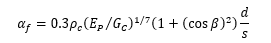top of page

# Elastic analysis mode in the PileGroup program

In our recent release of PileGroup Version 2.3.1, we have included a new elastic analysis module that performs a linear analysis based on elastic solutions. This blog post will give you a detailed explanation of this module. It is a highly convenient tool for a rapid analysis of pile groups that consist of a large number of piles.

In this analysis module, the approximate closed form solutions for single piles subject to axial loading (Randolph and Wroth 1978a and 1978b), torsion loading (Randolph 1981a) and lateral loading (Randolph 1981b) are incorporated into three-dimensional pile group analysis formulation of the program.

The soil is modelled as a linear elastic material with homogeneous stiffness, or the stiffness varies linearly with depth. Interaction factors between pile heads are derived from simple analytical formulas.

### 1. Elastic solutions to axially loaded single pile

The approximate elastic solution to axially loaded single pile has been developed by Randolph and Wroth (1978a and 1978b) and is given by the equation below:### 2. Elastic solutions to laterally loaded piles

For laterally loaded single piles, the lateral deflection and rotation at the pile head are calculated based on the following equations proposed by Randolph (1981a):where H and M are the horizontal force and bending moment acting at the pile head.For single pile with torsional loading applied at the pile head, the torque T and the angle of twist at the pile top can be expressed by the following equation (Randolph 1981b) for intermediate pile length:### 4 Interaction factors for laterally loaded piles

The interaction factors for laterally loaded piles with rigid pile head (restrained against rotation) are estimated based on the following equation proposed by Randolph (1981a):The interaction factor (deflection due to pile head horizontal force) is calculated as below:The interaction factor (deflection due to bending moment at the pile head) is calculated as:The interaction factor (rotation due to horizontal force at the pile head) is calculated as:The interaction factor (rotation due to bending moment at the pile head) is calculated as:### 5 Interaction factors for axially loaded piles

The interaction factors for axially loaded piles within the group are estimated by the following equation proposed by (Mylonakis and Gazetas ,1998):### 6 Example of linear analysis for pile group under vertical load

The example of a 3 x 3 pile group in Basile (2020) is analyzed using the analysis mode of linear analysis based on elastic solutions in the PileGroup program. In this example, the piles are constructed in a homogeneous elastic soil layer with a Young’s modulus of 50 MPa and a Poisson’s ratio of 0.5. The Young’s modulus of the pile material is 30 GPa. The pile length is 20 m and the pile diameter is 1 m. The spacing between the piles is 3 m. The pile group layout is shown in the figure below.Figure 1 Pile group layout

The pile cap is assumed to be rigid and the connection between the pile heads and pile cap is fully rigid (no rotation is allowed). A vertical load of 9 MN is applied in the center of the pile cap. The input soil parameters are shown in Figure 2.Figure 2 Soil material input parameters

Basile (2020) used this example to compare the results from the program PGROUPN in REPUTE with PIGLET (Randolph 2004) and DEFPIG (Poulos 1990). Figure 3 shows the pile axial forces within the pile group from the analysis using the PileGroup program. The maximum pile axial force is about 1313 kN. The ratio between the maximum and average pile axial force is about 1.31.Figure 3 Pile axial force results

The pile settlement results from the PileGroup program are shown in Figure 4. The pile settlement is about 7.4 mm for the vertical load of 9 MN.Figure 4 Pile settlement results

The comparison results from different programs are shown in the following table. It can be seen that the results from the PileGroup program are very close and practically identical to those calculated by REPUTE, PIGLET and DEFPIG programs.### References

Basile, F. (2020). Influence of cap rigidity on pile group design. Piling 2020: Proceeding of the Piling 2020 Conference, ICE Publishing.

Randolph, M. F. (1981). Analysis of the behaviour of piles subjected to torsion. Journal of Geotechnical Engineering Division, ASCE, 107(GT8), 1095-1111.

Randolph, M. F. (1981). The response of flexible piles to lateral loading. Geotechnique, 31(2), 247-259.

Randolph, M. F., and Wroth, C. P. (1978). Analysis of deformation of vertically loaded piles. Journal of Geotechnical Engineering Division, ASCE, 104(GT12), 1465-1488.

541 views